Function Repository Resource:

# UnwindingNumber

Evaluate the unwinding number

Contributed by: Jan Mangaldan
 ResourceFunction["UnwindingNumber"][z] gives the unwinding number 𝒰(z).

## Details

Mathematical function, suitable for both symbolic and numerical manipulation.
The unwinding number is defined by the relation z=log ez+2πi𝒰​(z).
ResourceFunction["UnwindingNumber"][z] returns an integer when z is any numeric quantity, whether or not it is an explicit number.
For exact numeric quantities, ResourceFunction["UnwindingNumber"] internally uses numerical approximations to establish its result. This process can be affected by the setting of the global variable \$MaxExtraPrecision.

## Examples

### Basic Examples (2)

Evaluate numerically:

 In:=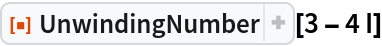Out=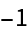Plot of the unwinding number in the complex plane:

 In:=Out=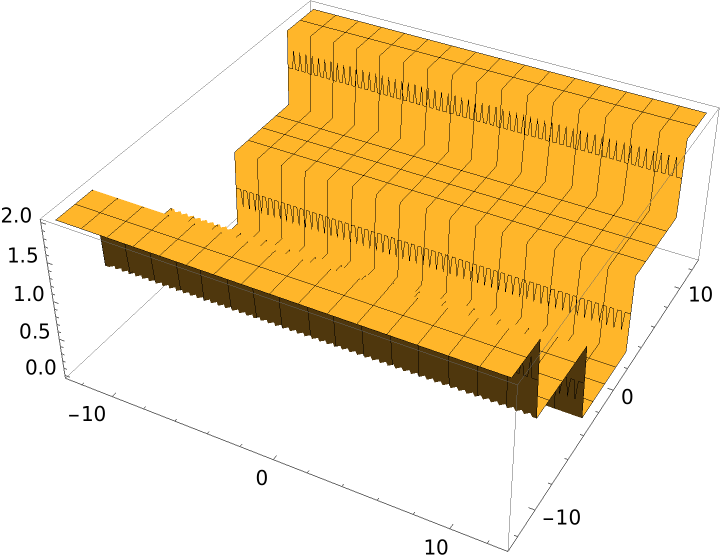### Scope (4)

Evaluate the unwinding number of a Root object:

 In:=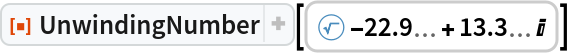Out=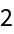Evaluate the unwinding number of a machine precision number:

 In:=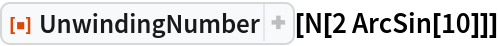Out=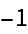Evaluate the unwinding number of an arbitrary precision number:

 In:=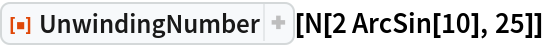Out=In:=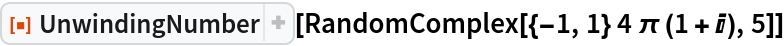Out=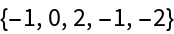### Applications (3)

The identity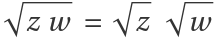does not generally hold for complex z and w:

 In:=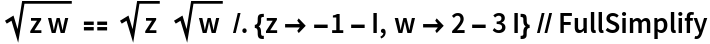Out=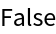Use the unwinding number to construct a formula that is valid in the entire complex plane:

 In:=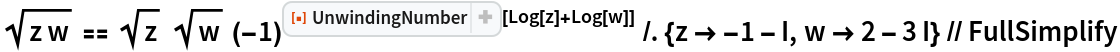Out=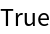The identity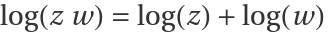does not generally hold for complex z and w:

 In:=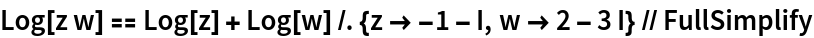Out=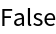Use the unwinding number to construct a formula that is valid in the entire complex plane:

 In:=Out=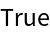A relationship between the inverse sine and the inverse tangent:

 In:=Out=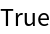### Properties and Relations (2)

The unwinding number is an integer:

 In:=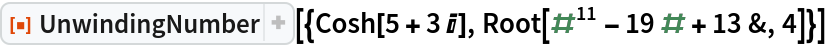Out=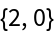Compare UnwindingNumber with one of its definitions:

 In:=Out=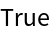### Possible Issues (2)

Numerical decision procedures with default settings cannot automatically resolve this value:

 In:=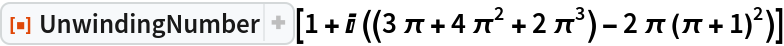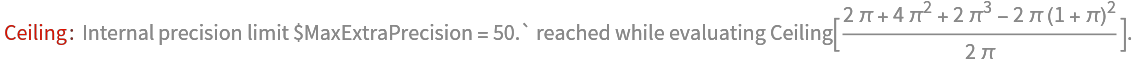Out=Use Simplify to resolve:

 In:=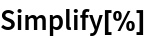Out=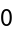### Neat Examples (2)

Define the Wright omega function:

 In:=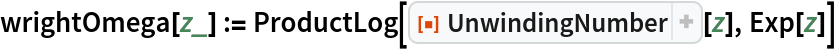Visualize the fringing fields of a semi-infinite parallel plate capacitor:

 In:=Out=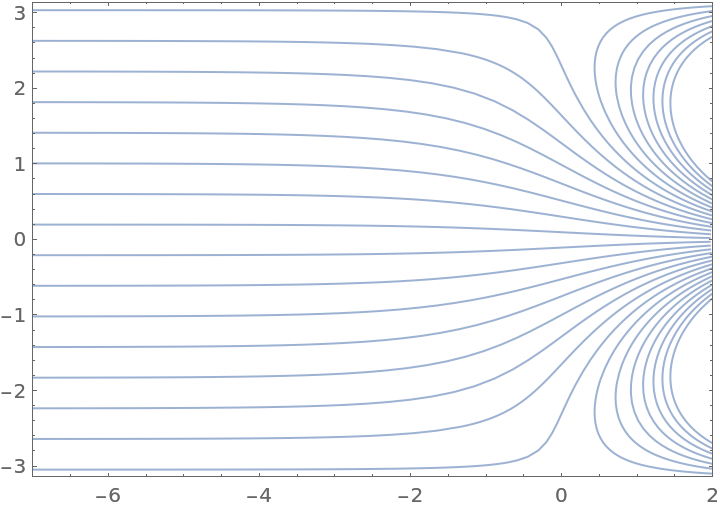## Version History

• 1.0.0 – 15 March 2021